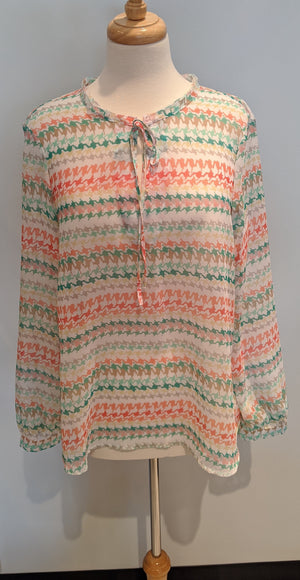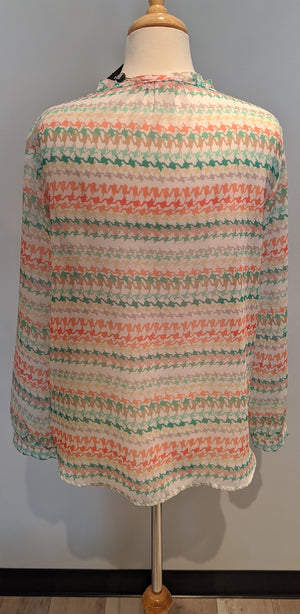Riani

# Print Blouse

Regular price \$345 Unit price  per

Shipping calculated at checkout.
• 100% polyester
• Machine wash cold. Hang to dry
• Tie on neckline
• Long sleeve
• Fits loose
• Easy wear

Size Guide

• 34 = 4 = XS
• 36 = 6 = S
• 38 = 8 = M
• 40 = 10 = M
• 42 = 12 = L
• 44 = 14 = L
• 46 = 16 = XL